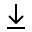MathBin Circle Member

A circle is a one-dimensional object that exists in two dimensions or higher. A circle is the boundary of a disk, so it has length (its circumference), but not area. The following equation gives a circle in two-dimensions with center (h, k) and radius r.

(xh)2 + (yk)2 = r2

The circumference of a circle is 2πr, and the area contained within the circumference (the area of the disk) is πr2.

The easiest way to describe a circle in two dimensions is to know the circle's center point and radius, where the radius is the distance from the center to the points along the circumference of the circle. But if you know the center and a point on the circumference, you can calculate the radius as the distance between the center and that point.

Somewhat harder is to find a circle given by three points. This involves solving a system of three equations for the circle's center and radius. There is no circle if the points are collinear (all on the same line). A unique circle is not determined by two points on its circumference unless they are the end points of its diameter.

A circle can be found given by its center and a line tangent to the circle's circumference. The trick is to find the point where the tangent line touches the circumference.

Bins in Two Dimensions